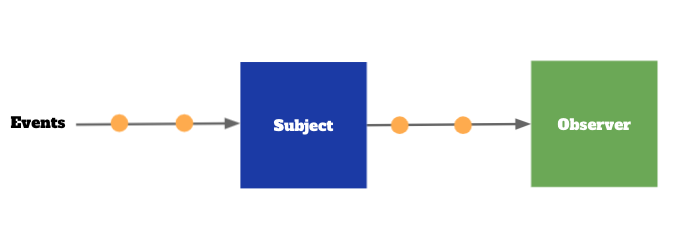DAY 8
1
Mobile Development

Observable Creation

Observable.just

val a = Observable.just(1, 2, 4)
a.subscribe { number -> println(number) }

// 1
// 2
// 4

Observable.create

val a = Observable.create<Int> { emitter: ObservableEmitter ->
println("start")
emitter.onNext(1)
emitter.onNext(2)
emitter.onComplete()
emitter.onNext(4) // This one never be sent
println("end")
}
println("before subscribe")
a.subscribe { number -> println(number) }

// before subscribe
// start
// 1
// 2
// end

Basic operation

map

val a = Observable.just(1, 2, 4)
val b = a.map { it * 2 }
b.subscribe { number -> println(number) }

// 2
// 4
// 8

filter

val a = Observable.just(1, 2, 4)
val b = a.filter { it % 2 == 0 }
val c = a.filter { it % 2 != 0 }
println("subscribe b")
b.subscribe { number -> println(number) }
println("subscribe c")
c.subscribe { number -> println(number) }

// subscribe b
// 2
// 4
// subscribe c
// 1

reduce

val a = Observable.just(1, 2, 4)
val b = a.reduce { t1, t2 ->
println("t1: \$t1, t2: \$t2")
t1 * t2
}

b.subscribe { number -> println("result: \$number") }

// t1: 1, t2: 2
// t1: 2, t2: 4
// result: 8

scan

val a = Observable.just(1, 2, 4)
val b = a.scan { t1, t2 ->
println("t1: \$t1, t2: \$t2")
t1 * t2
}

b.subscribe { number -> println("result: \$number") }

// result: 1
// t1: 1, t2: 2
// result: 2
// t1: 2, t2: 4
// result: 8

Subject

Subject 一種比較特別的 Observable，他同時是 Observable 也是 Observer，也就是說他可以透過 subscribe 其他 Observable 來獲得資料：

val a = BehaviorSubject.create<Int>()// BehaviorSubject 是其中一種 Subject 的實作
val b = Observable.just(1, 2, 3)

a.subscribe { number -> println("result: \$number") }
b.subscribe(a)

// result: 1
// result: 2
// result: 3val a = BehaviorSubject.create<Int>()

a.subscribe { number -> println("result: \$number") }

a.onNext(1)
a.onNext(2)
a.onComplete()
a.onNext(4)

// result: 1
// result: 2

三種不同類型的 Subject

• BehaviorSubject：不管是任何 Observer 在任何時間 subscribe，一開始都會收到最新的一筆資料，跟 LiveData 的行為一樣。
• PublishSubject：永遠只會收到當下傳送的資料，在 subscribe 之前的任何資料都是不會收到的。
• ReplaySubject：記得所有傳輸過的資料，每一個新的 Observer 都會完整的收到歷史資料，但是使用上要特別注意，記憶體的量不是無限的！

val behavior = BehaviorSubject.create<Int>()
val publish = PublishSubject.create<Int>()
val replay = ReplaySubject.create<Int>()

behavior.onNext(1)
publish.onNext(1)
replay.onNext(1)
behavior.onNext(2)
publish.onNext(2)
replay.onNext(2)

behavior.subscribe { number -> println("behavior: \$number") }
publish.subscribe { number -> println("publish: \$number") }
replay.subscribe { number -> println("replay: \$number") }

behavior.onNext(4)
publish.onNext(4)
replay.onNext(4)

// behavior: 2
// replay: 1
// replay: 2
// behavior: 4
// publish: 4
// replay: 4

Merge Observable

Observable 有很多不一樣的組合方式，在這裡只會提到幾個常用的，跟本專案有用到的其中幾個，其他就要靠讀者自己看文件了。

CombineLatest

val a = PublishSubject.create<Int>()
val b = PublishSubject.create<Int>()

Observables.combineLatest(a, b)
.map { (first, second) ->
println("first: \$first, second: \$second")
first + second
}
.subscribe { number -> println("result: \$number") }

a.onNext(1)
a.onNext(2)
b.onNext(1)
b.onNext(3)
a.onNext(5)
b.onNext(8)

// first: 2, second: 1
// result: 3
// first: 2, second: 3
// result: 5
// first: 5, second: 3
// result: 8
// first: 5, second: 8
// result: 13

Zip

val a = PublishSubject.create<Int>()
val b = PublishSubject.create<Int>()

Observables.zip(a, b)
.map { (first, second) ->
println("first: \$first, second: \$second")
first + second
}
.subscribe { number -> println("result: \$number") }

a.onNext(1) //  []
a.onNext(2) // [1, 2] []
b.onNext(1) //  [] emit (1, 1)
b.onNext(3) // [] [] emit (2, 3)
a.onNext(5) //  []
b.onNext(8) // [] [] emit (5, 8)

// first: 1, second: 1
// result: 2
// first: 2, second: 3
// result: 5
// first: 5, second: 8
// result: 13

withLatestFrom

val a = PublishSubject.create<Int>()
val b = PublishSubject.create<Int>()

a.withLatestFrom(b) { first, second ->
println("first: \$first, second: \$second")
first + second
}
.subscribe { number -> println("result: \$number") }

a.onNext(1) // b 沒有值，所以不會觸發
a.onNext(2) // b 沒有值，所以不會觸發
b.onNext(1) // b 有值了，但是主動權是在 a 身上，所以一樣不會觸發
a.onNext(3) // b 有值了，由 a 主動觸發，得到 3+1 = 4
a.onNext(5) // b 有值了，由 a 主動觸發，而且會拿到一樣的 b，得到 5+1 = 6
b.onNext(8) // 只是更新 b ，不會有新的資訊產生出來

// first: 3, second: 1
// result: 4
// first: 5, second: 1
// result: 6

Optional

fun main() {
val a: String? = null
val b: String? = "Hihi"
val optA = Optional.ofNullable(a)
val optB = Optional.ofNullable(b)

printOptStringLength(optA)
printOptStringLength(optB)
}

private fun printOptStringLength(optString: Optional<String>) {
optString.map { it.length }.ifPresent { length ->
println("length: \$length")
}
}

a 跟 b 的型別一樣都是 nullable ，如果我現在有個需求是想知道這字串的長度，要怎麼做呢？使用 Kotlin 的作法可能是用 ?. 這種語法來做安全的呼叫，但是使用 Optional 的話，就可以去除掉使用 ?. 這樣的語法，取而代之的是 map

fun main() {
multiplyNumberAndStringLen(4, null)
multiplyNumberAndStringLen(null, "Hihi")
multiplyNumberAndStringLen(4, "Hihi")
}

private fun multiplyNumberAndStringLen(a: Int?, b: String?) {
val optA = Optional.ofNullable(a)
val optB = Optional.ofNullable(b)

optA.flatMap { number -> optB.map { str -> number * str.length } }
.ifPresent { number ->
println("Multiply result: \$number")
}
}

// Multiply result: 16

BoardViewModel moveNote 的實作

fun moveNote(noteId: String, delta: Position) {
Observable.just(Pair(noteId, delta))
.withLatestFrom(allNotes) { (noteId, delta), notes ->
val currentNote = notes.find { it.id == noteId }
Optional.ofNullable(currentNote?.copy(position = currentNote.position + delta))
}
.mapOptional { it }
.subscribe { newNote ->
noteRepository.putNote(newNote)
}
}

Observable.combineLatest(Observable.just(Pair(noteId, delta)), allNotes)

NoteRepository putNote 的實作

class InMemoryNoteRepository(): NoteRepository {

private val notesSubject = BehaviorSubject.create<List<Note>>()
private val noteMap = ConcurrentHashMap<String, Note>()

init {
val initNote = Note.createRandomNote()
noteMap[initNote.id] = initNote
notesSubject.onNext(noteMap.elements().toList())
}

override fun getAll(): Observable<List<Note>> {
return notesSubject.hide()
}

override fun putNote(newNote: Note) {
noteMap[newNote.id] = newNote
notesSubject.onNext(noteMap.elements().toList())
}
}